# Regex Match in Excel: regular expression matching with examples

In this tutorial, we'll have an in-depth look at how to use regex to match strings in Excel.

When you need to find a certain value in a range of cells, you'd use the MATCH function. When looking for a specific string in a cell, the FIND and SEARCH functions come in handy. And how do you know if a cell contains information that matches a given pattern? Obviously, by using regular expressions. But Excel does not support regexes! No worries, we'll force it to :)

## Excel VBA Regex function to match strings

As it's pretty clear from the heading, in order to use regular expressions in Excel, you need to create your own function. Luckily, Excel's VBA has an inbuilt RegExp object, which you can use in your code like shown below:

```Public Function RegExpMatch(input_range As Range, pattern As String, Optional match_case As Boolean = True) As Variant
Dim arRes() As Variant 'array to store the results
Dim iInputCurRow, iInputCurCol, cntInputRows, cntInputCols As Long 'index of the current row in the source range, index of the current column in the source range, count of rows, count of columns

On Error GoTo ErrHandl

RegExpMatch = arRes

Set regex = CreateObject("VBScript.RegExp")
regex.pattern = pattern
regex.Global = True
regex.MultiLine = True
If True = match_case Then
regex.ignorecase = False
Else
regex.ignorecase = True
End If

cntInputRows = input_range.Rows.Count
cntInputCols = input_range.Columns.Count
ReDim arRes(1 To cntInputRows, 1 To cntInputCols)

For iInputCurRow = 1 To cntInputRows
For iInputCurCol = 1 To cntInputCols
arRes(iInputCurRow, iInputCurCol) = regex.Test(input_range.Cells(iInputCurRow, iInputCurCol).Value)
Next
Next

RegExpMatch = arRes
Exit Function
ErrHandl:
RegExpMatch = CVErr(xlErrValue)
End Function
```

Paste the code in the VBA editor, and your new RegExpMatch function is ready for use. If you are not very experienced with VBA, this guide may be helpful: How to insert VBA code in Excel.

Note. After inserting the code, remember to save your file as a macro-enabled workbook (.xlsm).

### RegExpMatch syntax

The RegExpMatch function checks whether any part of the source string matches a regular expression. The result is a Boolean value: TRUE if at least one match is found, FALSE otherwise.

Our custom function has 3 arguments - the first two are required and the last one is optional:

RegExpMatch(text, pattern, [match_case])

Where:

• Text(required) - one or more strings to search in. Can be supplied as a cell or range reference.
• Pattern(required) - the regular expression to match. When placed directly in a formula, a pattern must be enclosed in double quotes.
• Match_case (optional) - defines the match type. If TRUE or omitted (default), case-sensitive matching is performed; if FALSE - case-insensitive.

The function works in all versions of Excel 365, Excel 2021, Excel 2019, Excel 2016, Excel 2013 and Excel 2010.

### 3 things you should know about RegExpMatch

Before we get to practical calculations, please take notice of the following points that clarify some technicalities:

1. The function can process a single cell or range of cells. In the latter case, the results are returned in the neighboring cells in the form of a dynamic array, or spill range, like shown in this example.
2. By default, the function is case-sensitive. To ignore text case, set the match_case argument to FALSE. Because of the VBA Regexp limitations, the case-insensitive pattern (?i) is not supported.
3. If a valid pattern is not found, the function returns FALSE; if the pattern is invalid, a #VALUE! error occurs.

Below, you'll find a few regex match examples that were created for demonstration purposes. We cannot guarantee that our patterns will work faultlessly with a wider range of input data in your real worksheets. Before putting in production, be sure to test and adjust our samples patterns according to your needs.

## How to use regex to match strings in Excel

When all the strings you want to match have the same pattern, regular expressions are an ideal solution.

Supposing you have a range of cells (A5:A9) containing various details about some items. You wish to know which cells have SKUs. Assuming that each SKU consists of 2 capital letters, a hyphen, and 3 digits, you can match them using the following expression.

Pattern: \b[A-Z]{2}-\d{3}\b

Where [A-Z]{2} means any 2 uppercase letters from A to Z and \d{3} means any 3 digits from 0 to 9. The \b character denotes a word boundary, meaning an SKU is a separate word, and not part of a bigger string such as 23-MAR-2022.

With the pattern established, we can move on to writing a formula. Essentially, using a custom function is no different from a native one. As soon as you start typing a formula, the function's name will appear in the list suggested by Excel's AutoComplete. However, there are a couple of nuances in Dynamic Array Excel (Microsoft 365) and traditional Excel (2019 and older versions).

### Match string in one cell

To match a string in a single cell, refer to that cell in the first argument. The second argument is supposed to contain a regular expression.

`=RegExpMatch(A5, "\b[A-Z]{2}-\d{3}\b")`

The pattern can also be kept in a predefined cell, which is locked with an absolute reference (\$A\$2):

`=RegExpMatch(A5, \$A\$2)`

After entering the formula in the first cell, you can drag it down to all other rows.

This method works beautifully in all Excel versions.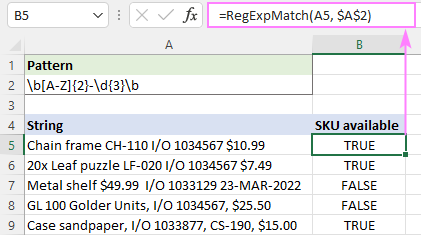### Match strings in multiple cells at once

To match multiple strings with a single formula, include a range reference in the first argument:

`=RegExpMatch(A5:A9, "\b[A-Z]{2}-\d{3}\b")`

In Excel 365 that supports dynamic arrays, it works this way - you type the formula in the first cell, hit Enter, and the formula automatically spills into the below cells.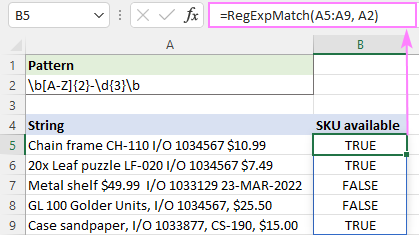In Excel 2019 and earlier, it only works as a traditional CSE array formula, which is entered in a range of cells and completed by pressing the Ctrl + Shift + Enter keys together.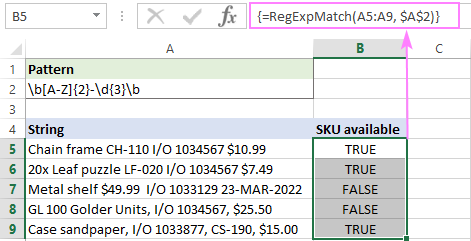## Regex to match number

To match any single digit from 0 to 9, use the \d character in the regex. Depending on your particular task, add a suitable quantifier or create a more complex pattern.

### Regex to match any number

To match any number of any length, put the + quantifier right after the /d character, which says to look for numbers containing 1 or more digits.

Pattern: \d+

`=RegExpMatch(A5:A9, "\d+")`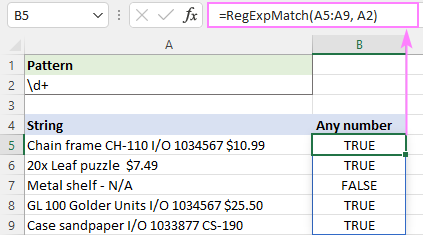### Regex to match number of specific length

If your goal is to match numeric values containing a certain number of digits, then use \d together with an appropriate quantifier.

For example, to match invoice numbers consisting of exactly 7 digits, you'd use \d{7}. However, please keep in mind that it will match 7 digits anywhere in the string including a 10-digit or 100-digit number. If this is not what you are looking for, put the word boundary \b on both sides.

Pattern: \b\d{7}\b

`=RegExpMatch(A5:A9, "\b\d{7}\b")`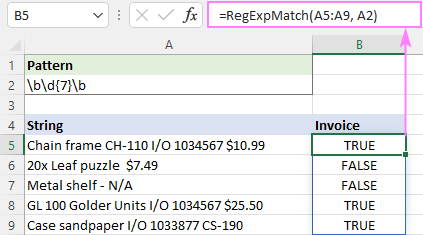## Regex to match phone numbers

Since phone numbers can be written in various formats, matching them requires a more sophisticated regular expression.

In the below dataset, we will be searching for 10-digit numbers that have 3 digits in the first 2 groups and 4 digits in the last group. The groups can be separated with a period, hyphen or space. The first group may or may not be enclosed in parentheses.

Pattern: (\(\d{3}\)|\d{3})[-\.\s]?\d{3}[-\.\s]?\d{4}\b

Breaking down this regular expression, here's what we get:

• The first part (\(\d{3}\)|\d{3}) matches a 3-digit number either inside the parentheses or without parentheses.
• The [-\.\s]? part means 0 or 1 occurrence of any character in square brackets: hyphen, period, or whitespace.
• Next, there is one more group of 3 digits d{3} followed by any hyphen, period or whitespace [\-\.\s]? appearing 0 or 1 time.
• The last group of 4 digits \d{4} is followed by a word boundary \b to make it clear that a phone number cannot be part of a bigger number.

With the original string in A5 and the regular expression in A2, the formula takes this form:

`=RegExpMatch(A5, \$A\$2)`

… and works exactly as expected: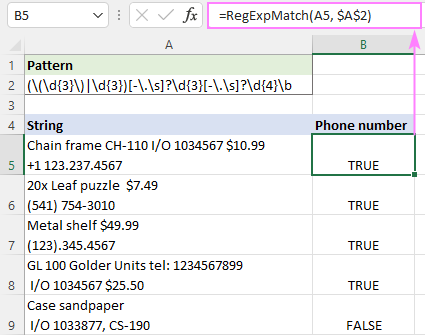Notes:

• International codes are not checked, so they may or may not be present.
• In regular expressions, \s stands for any whitespace character such as a space, tab, carriage return, or new line. To allow only spaces, use [-\. ] instead of [-\.\s].

## Regex to NOT match character

To find strings that do NOT contain a certain character, you can use negated character classes [^ ] that match anything NOT in brackets. For example:

• [^13] will match any single character that is not 1 or 3.
• [^1-3] will match any single character that is not 1, 2 or 3 (i.e. any digit from 1 to 3).

In a list of phone numbers, suppose you want to find those that do not have a country code. Keeping in mind that any international code includes the + sign, you can use the [^\+] character class to find strings that do not contain a plus sign. It is important to realize that the above expression matches any single character that is not +. Because a phone number can be anywhere in a string, not necessarily in the very beginning, the * quantifier is added to check each subsequent character. The start ^ and end \$ anchors ensure that the entire string is processed. As the result, we get the below regular expression that says "don't match the + character in any position in the string".

Pattern: ^[^\+]*\$

`=RegExpMatch(A5, "^[^\+]*\$")`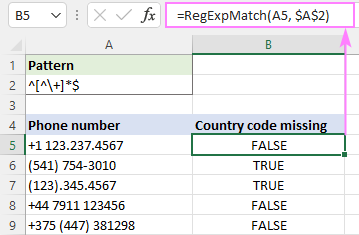## Regex to NOT match string

Though there is no special regular expression syntax for not matching a specific string, you can emulate this behavior by using a negative lookahead.

Supposing you wish to find strings that do not contain the word "lemons". This regular expression will work a treat:

Pattern: ^((?!lemons).)*\$

Obviously, some explanation is needed here, right? The negative lookahead (?!lemons) looks to the right to see if there's no word "lemons" ahead. If "lemons" is not there, then the dot matches any character except a line break. The above expression performs just one check, and the * quantifier repeats it zero or more times, from the start of the string anchored by ^ to the end of the string anchored by \$.

To ignore the text case, we set the 3th argument to FALSE to make our function case-insensitive:

`=RegExpMatch(A5, \$A\$2, FALSE)`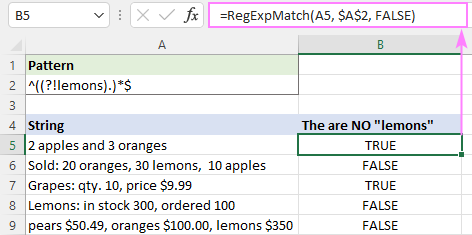Tips and notes:

• The above regex only works for single-line strings. In case of milti-line strings, the ^ and \$ characters match the beginning and end of each line instead of the beginning and end of the input string, therefore the regex only searches in the first line.
• To match strings that do not start with certain text, use a regular expression such as ^(?!lemons).*\$
• To match strings that do not end with certain text, include the end string anchor into the search pattern: ^((?!lemons\$).)*\$

## Case insensitive matching

In classic regular expressions, there is a special pattern for case-insensitive matching (?i), which is not supported in VBA RegExp. To overcome this limitation, our custom function accepts the 3rd optional argument named match_case. To do case-insensitive matching, simply set it to FALSE.

Let's say you wish to identify dates such as 1-Mar-22 or 01-MAR-2022. To match the dd-mmm-yyyy and d-mmm-yy formats, we are using the following regular expression.

Pattern: \b\d{1,2}-(Jan|Feb|Mar|Apr|May|Jun|Jul|Aug|Sep|Oct|Nov|Dec)-(\d{4}|\d{2})\b

Our expression searches for a group of 1 or 2 digits, followed by a hyphen, followed by any of the month abbreviations separated by | which enables the OR logic, followed by a group of 4 or 2 digits.

Why not use a simpler pattern such as \d{1,2}-[A-Za-z]{3}-\d{2,4}\b? To prevent false positive matches like 01-ABC-2020.

Enter the pattern in A2, and you'll get the following formula:

`=RegExpMatch(A5, \$A\$2, FALSE)`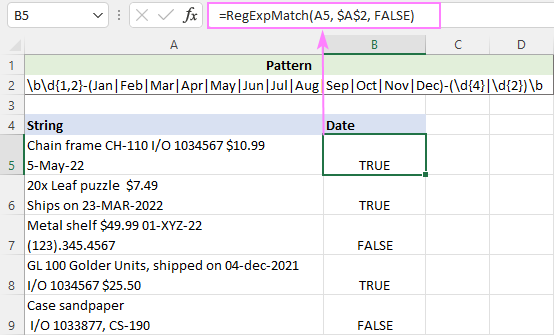## Regex to match valid email addresses

As generally known, an email address consists of 4 parts: username, @ symbol, domain name (mail server) and top-level domain (such as .com, .edu, .org, etc.). To check the email address validity, we'll need to replicate the above structure using regular expressions.

Pattern: \b[\w\.\-]+@[A-Za-z0-9]+[A-Za-z0-9\.\-]*[A-Za-z0-9]+\.[A-Za-z]{2,24}\b

To better understand what's going on here, let's take a closer look at each part:

• Username may include letters, numbers, underscores, dots and hyphens. Keeping in mind that \w matches any letter, digit or underscore, we get the following regex: [\w\.\-]+
• Domain name may include uppercase and lowercase letters, digits, hyphens (but not in the first or last position) and dots (in case of subdomains). Since underscores are not allowed, instead of \w we are using 3 different character sets: [A-Za-z0-9]+[A-Za-z0-9\.\-]*[A-Za-z0-9]+
• Top-level domain consists of a dot followed by uppercase and lowercase letters. It can contain from 2 to 24 letters (the longest TLD currently in existence): \.[A-Za-z]{2,24}
Note. The pattern assumes the domain name contains 2 or more alphanumeric characters.

With the original text in A5 and the pattern in A5, the formula takes this shape:

`=RegExpMatch(A5, \$A\$2)`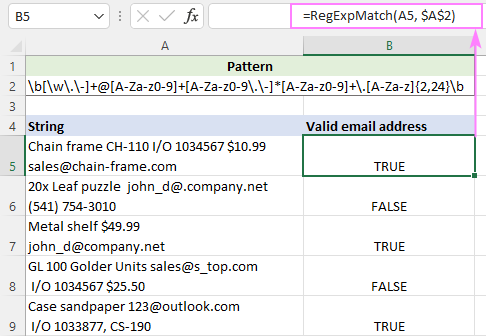Or you could use a simpler regular expression for email validation with either a lowercase or uppercase character set:

Pattern: \b[\w\.\-]+@[a-z0-9]+[a-z0-9\.\-]*[a-z0-9]+\.[a-z]{2,24}\b

`=RegExpMatch(A5, \$A\$2, FALSE)`

## Excel IF formula with match regex

Due to the fact that inbuilt and custom functions go along nicely, there is nothing that would prevent you from using them together in a single formula.

To return or calculate something if a regular expression is matched and something else if it's not matched, embed the custom RegExpMatch function in the logical text of IF:

IF(RegExpMatch(…), [value_if_true], [value_if_false])

For example, if a string in A5 contains a valid email address, you can return "Yes"; otherwise "No".

`=IF(RegExpMatch(A5, \$A\$2,), "Yes", "No")`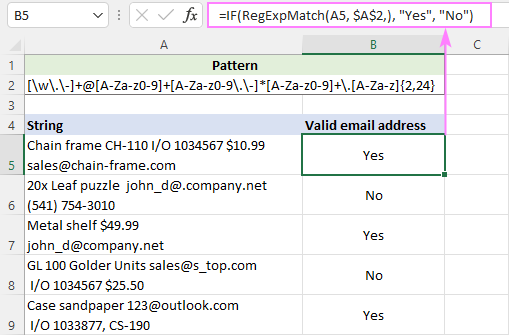## Count if regex is matched

Because native Excel functions don't support regular expressions, it's not possible to put a regex directly in the COUNTIS or COUNTIFS function. Luckily, you can emulate this functionality using our custom function.

Supposing you've used a regex to match phone numbers and output the results in column B. To find out how many cells contain phone numbers, you just need to count the TRUE values in B5:B9. And that can be easily done using the standard COUNTIF formula:

`=COUNTIF(B5:B9, TRUE)`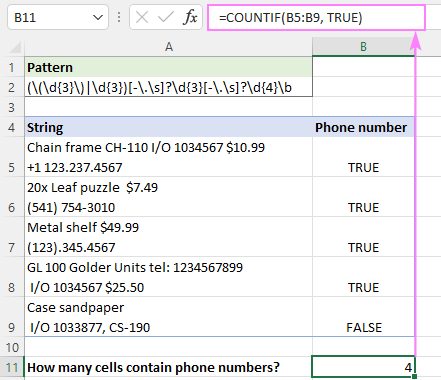Do not want any extra columns in your worksheet? No problem. Keeping in mind that our custom function can process multiple cells at a time and Excel's SUM can add up values in an array, here's what you do:

• Supply a range reference to RegExpMatch, so it returns an array of TRUE and FALSE values.
• Use a double negation (--) to coerce the logical values to ones and zeros.
• Get the SUM function to add up 1's and 0's in the resulting array.

`=SUM(--RegExpMatch(A5:A9, \$A\$2))`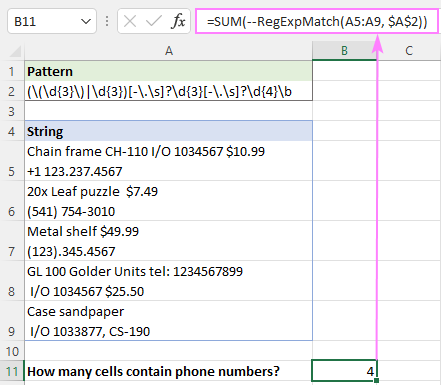## Regex matching with Ultimate Suite

The users of our Ultimate Suite can leverage four powerful Regex functions without adding any VBA code to their workbooks as they are smoothly integrated into Excel during the add-in installation. Our custom functions are processed by the standard .NET RegEx engine and support full-featured classic regular expressions.

### How to use custom RegExMatch function

Assuming you have the latest version of Ultimate Suite installed (2021.4 or later), you can create a Regex Match formula in two simple steps:

1. On the Ablebits Data tab, in the Text group, click Regex Tools.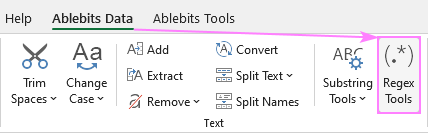2. On the Regex Tools pane, do the following:
• Select the source strings.
• Choose the Match option.
• To have the results as formulas, not values, select the Insert as a formula check box.
• Click the Match button.
•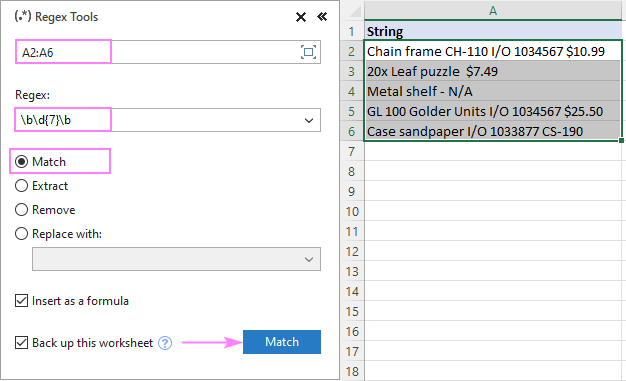A moment later, the AblebitsRegexMatch function is inserted in a new column to the right of your data.

In the screenshot below, the function checks whether the strings in column A contain 7-digit numbers or not.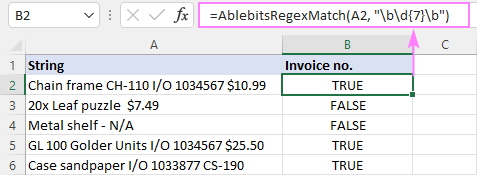Tips:

• The function can be inserted directly in a cell via the standard Insert Function dialog box, where it is categorized under AblebitsUDFs.
• By default, a regular expression is added to the formula, but you can also keep it in a separate cell. For this, just use a cell reference for the 2nd argument.
• By default, the function is case-sensitive. For case-insensitive matching, use the (?i) pattern.